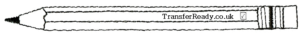# Calculating the Volume of Cubes and Cuboids

Volumes of cubes and cuboids is popular on the transfer tests. Calculate these by multiplying l x b x h.

Be careful with the units in this worksheet – where they are different, always change the given measurements into the unit used in the answer line.